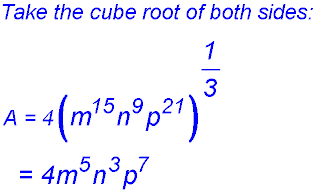Dr. J's Maths.com
Where the techniques of Maths
are explained in simple terms.

Algebra - Indices and Powers - additional basic questions.
Test Yourself 2 - Solutions.

The questions in this test required you to simplify the expressions so as to evaluate your understanding of the five basic concepts underlying this topic.

Lets see how you went with these slightly more difficult questions.

Fractions with Powers.

 1.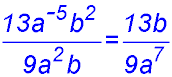2.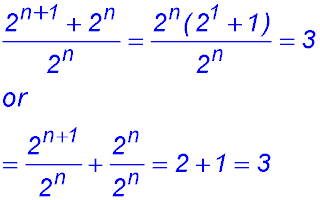3.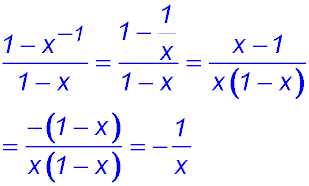4.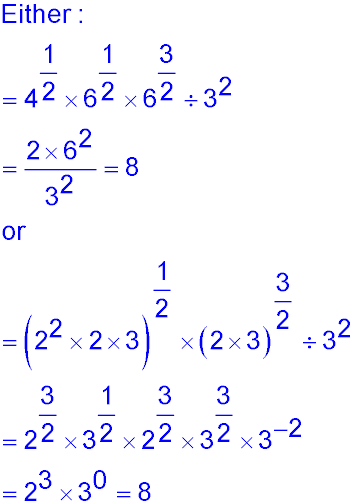5.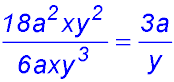6. Write the following fraction without using negative indices:7. Simplify:8.9.Powers of powers:

 10.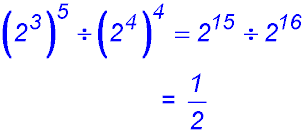11.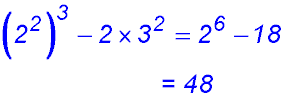12.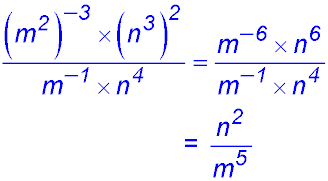13.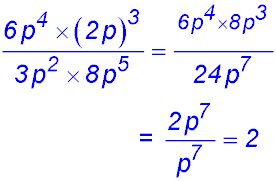14.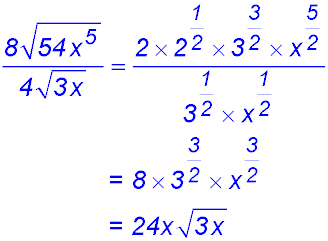15.Negative powers:

 16.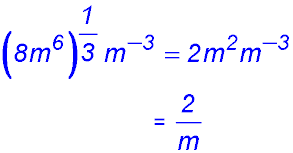17.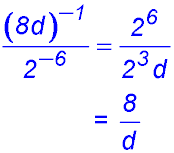18.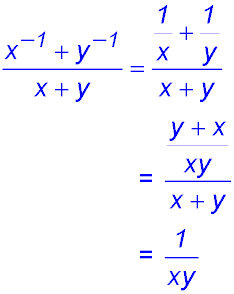19.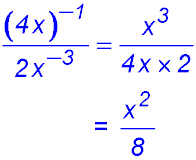20.21. With respect to the following numbers, 2-(½)k, 2(½)k, 2k, 2-k and given k is a positive number: specify the smallest number; specify the largest number. Is the order the same if k is a positive fraction? We can rewrite the four numbers as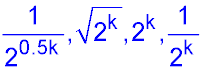. As k increases in value, k > 0.5k so dividing by the term in 2k (with the larger index in the denominator) results in a smaller result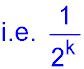. As a square root of a positive number larger than 1 is always less than the number itself, 2k must be the largest value.

Exponents of 1 or zero:

 22.23.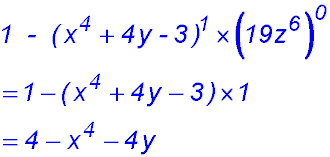24.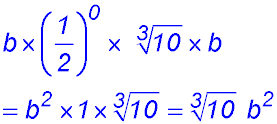Mixtures:

 25.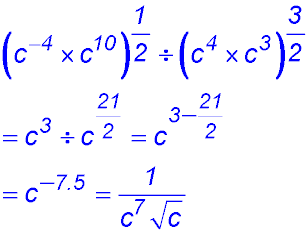26.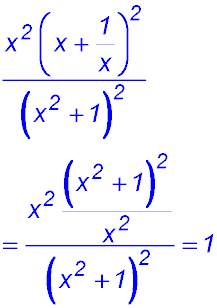27.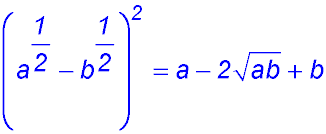28.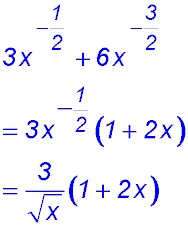29.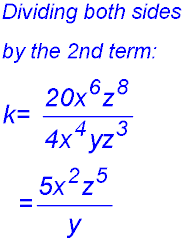30.